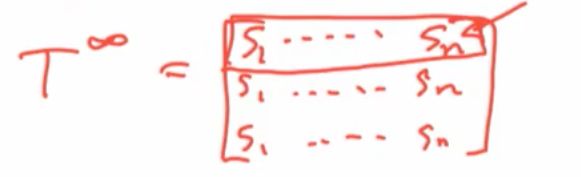# Outline: DeGroot Model¶

• Basic Definitions

• ==> When is there convergence?

• When is there a consensus?

• Who has influence?

• When is the consensus accurate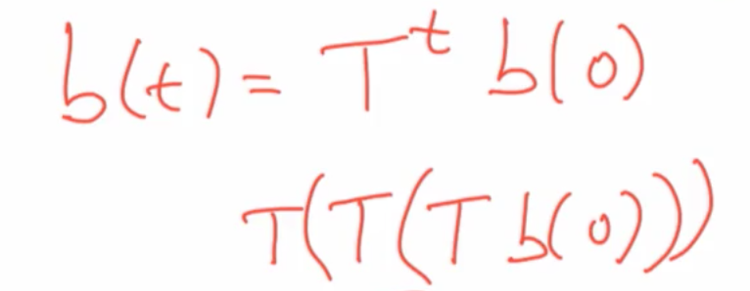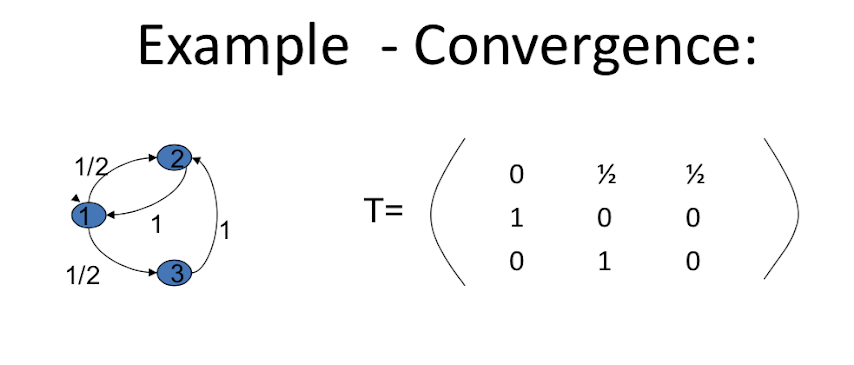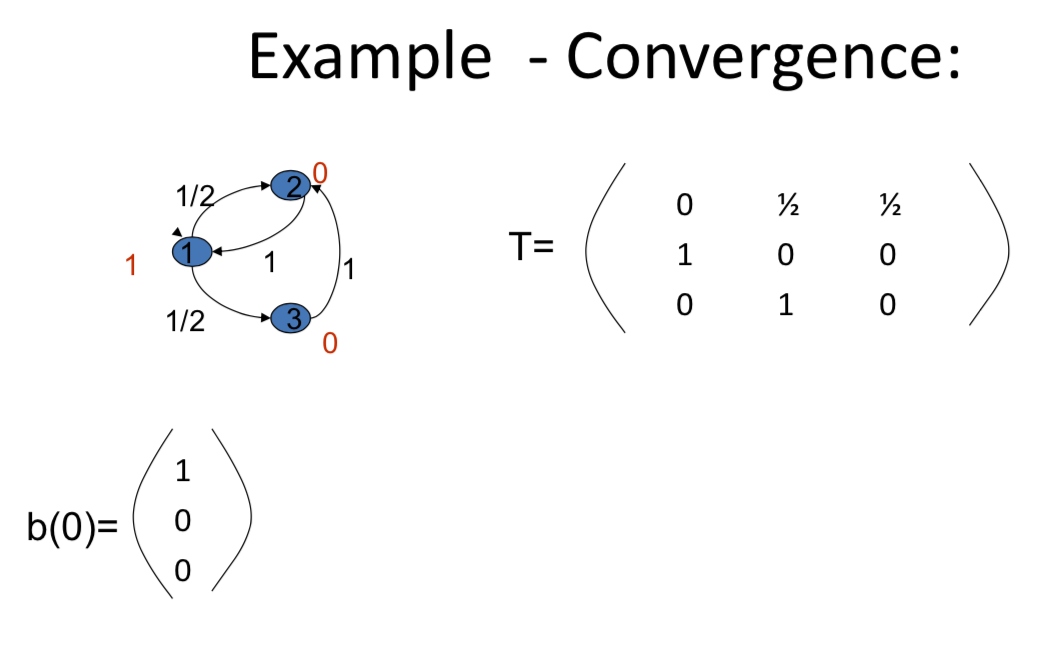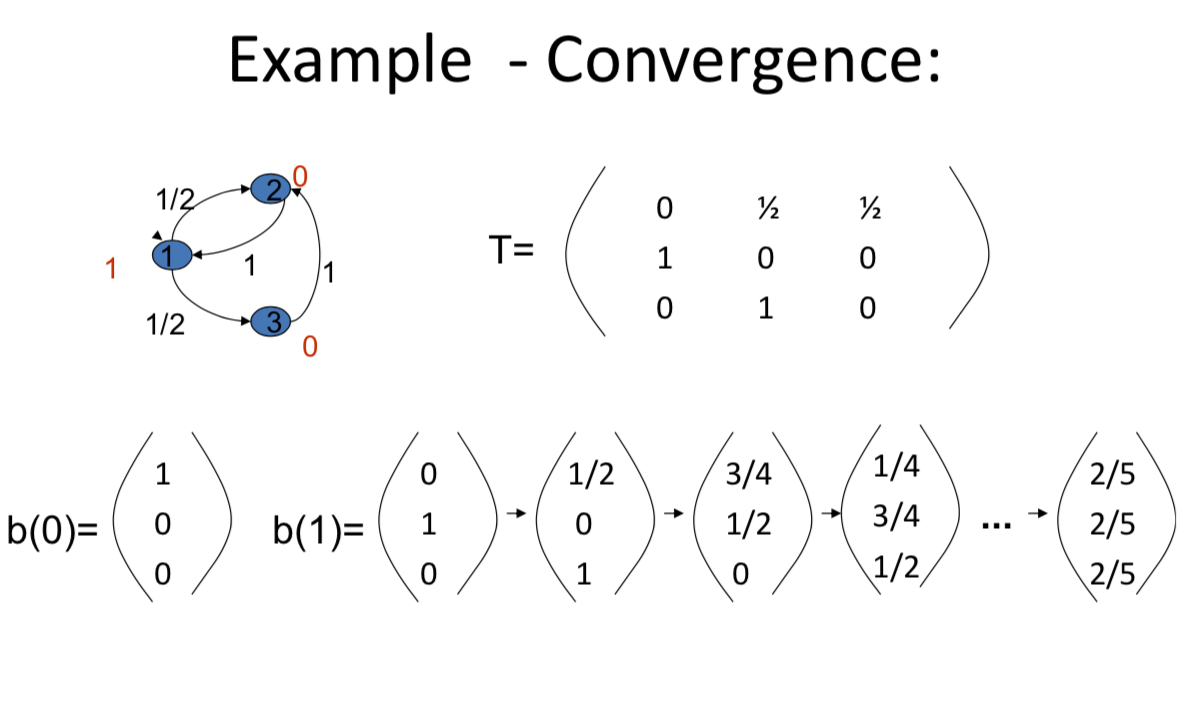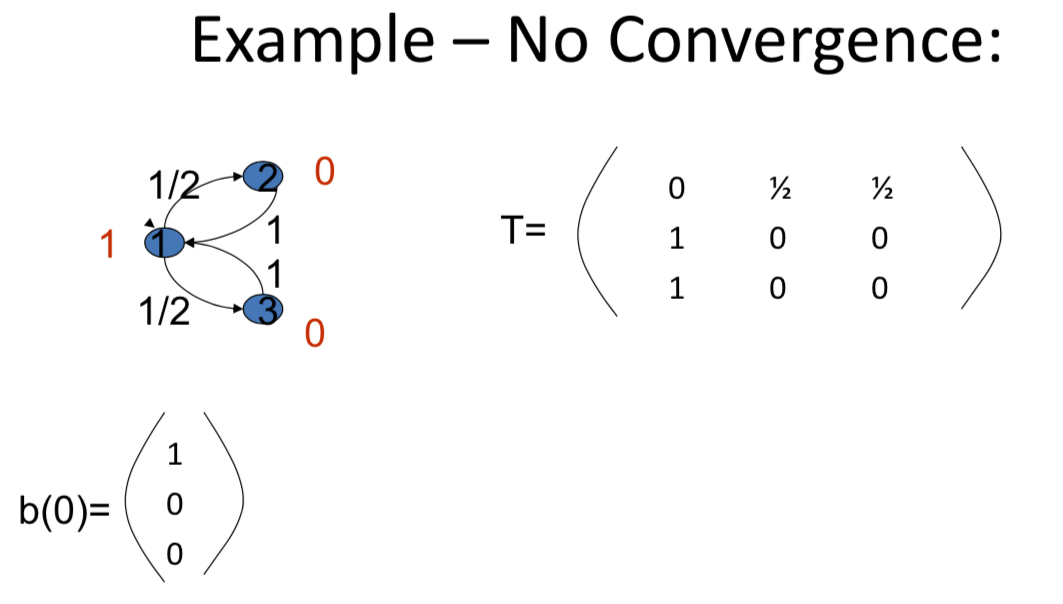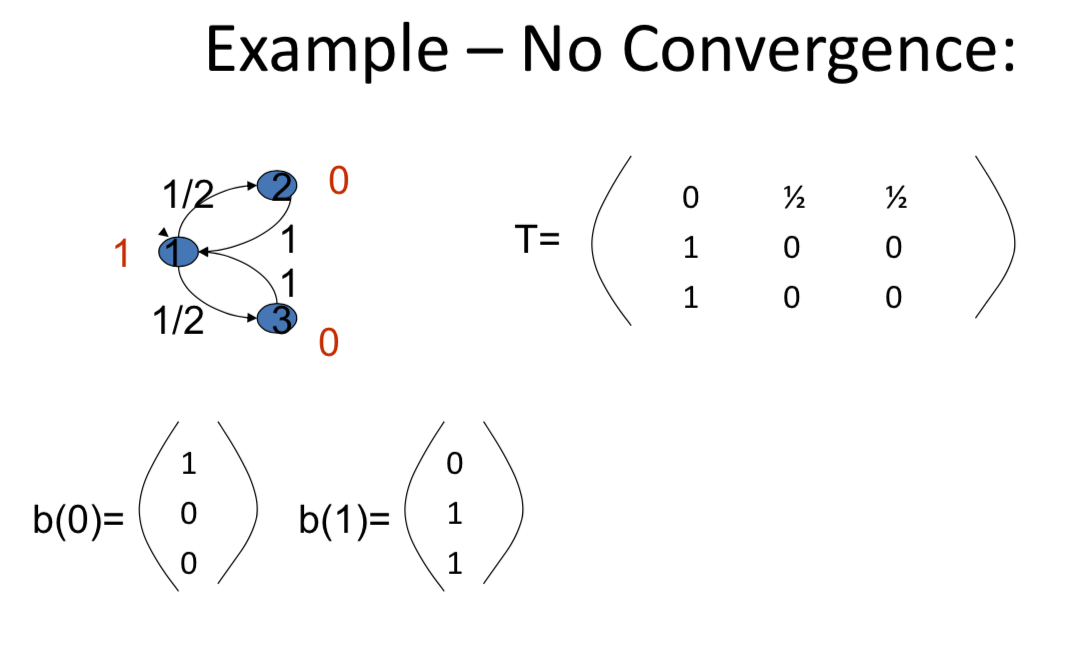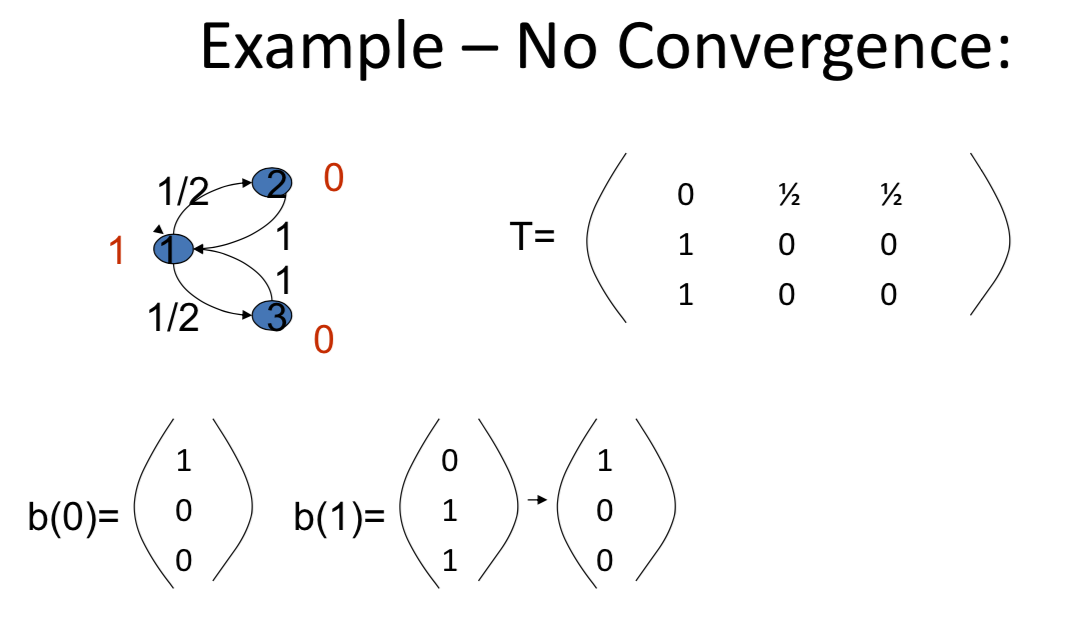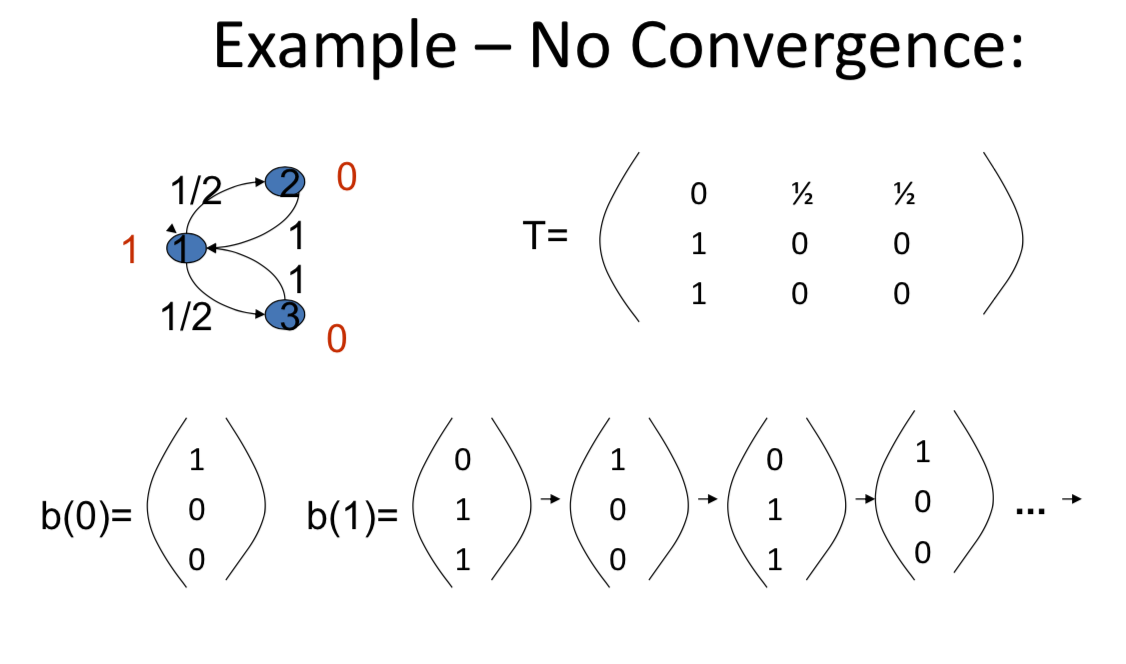Now what's true about this, in order for this to happen. it's actually based on the cycles in this graph and here all the cycles are of length two. And we've got the beliefs in, in such a way that they keep blinking back and forth, and so it's possible for the beliefs to keep switching back and forth on these cycles. And so it's there's a cyclicity in the graph which gets is possible to reproduce in the beliefs. Okay? Now, it could be that, you know, for other beliefs you would, might have converged. If we started everybody with a belief of 1, they would've converged to a belief of 1. So it depends on which things which we choose but whenever there are even cycles, all the cycles are even in, in a given graph, then we could find situations where we're going to get non-convergence. So more generally let's actually look at the

# Convergence¶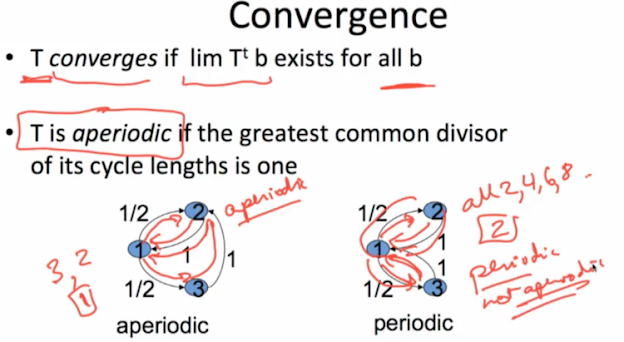One cycle lenght is "3", the other cycle length is "2", the greatest common divisor of "3" and "2" they don't divide into each other, the only thing that divides both those is "1", greatest common divisor is "1"

# Theorem¶

Suppose T is strongly connected

T is convergent if and only if it is aperiodic

• "strongly connected" is going to say that from every given individual, there exists a directed path from i to j.

$\exists$ directed path i to j $\forall i, j$

T is convergent if and only if: lim $T^t = (1,1,...,1)^T s$ where s is the unique lhs(left hand side) eigenvector with eigenvalue 1